# Multiplying Vectors I: The Scalar Product

Having covered the basics of defining and adding vectors, multiplying by scalars and finding unit vectors, it’s time to look at multiplying vectors together. What makes this entirely unlike working with numbers is that there are two ways (in fact, more than two!) to multiply two vectors. We’ll look at one of those today, the “dot product”, whose result is not a vector but a scalar (so that it is also called the “scalar product”, or sometimes the “inner product”). We’ll see two different definitions, and several different proofs of the key relationship, emphasizing different details.

## What is it? A choice of definitions

We’ve had a number of questions about what the dot product is, and have given almost contradictory answers, because it can be defined in two different ways. I’m going to try to put them together to make a coherent whole. Here is our first question, from 1998:

Explaining the Dot Product

I am currently a high school student teacher teaching trigonometry. We are doing a unit on vectors. When inner (dot) product was taught, many questions were raised. Everyone understood that when given vector u and vector v, the dot product is ||u|| times ||v|| times the cosine of the angle between them, but we had a problem when we got the answer. Everyone understood that the answer was a scalar, not a vector, but there is no graphical representation for what this scalar stands for.  I have checked numerous sources (every text book I could get my hands on, the internet, the math department at the university I am attending, and the math department where I am student teaching). I have had the students look for an answer on the internet and in the library. We did an activity drawing vectors and comparing the dot product with the vectors. None of us has been able to find an understandable meaning of dot product. We have exhausted our resources and hope you can help us. We have done problems involving work and the dot product, so we have seen a real world application, but we are still confused as to what it really is.

QUESTION:
Exactly what does the dot product represent? Is there a graphical explanation for the resulting scalar?

Please help us clear the confusion. Thank you.

Paul has been taught that the dot product of two vectors u and v is the product of their magnitudes and the cosine of the angle between them. To him, evidently, this doesn’t seem tangible enough, or important enough, to be its essence. What is it, really?

Doctor Anthony answered, changing the starting point:

You are perhaps thinking of dot products the wrong way around. The dot product of the vector (x1, y1, z1) and the vector (x2, y2, z2) is written down in a few seconds:

v1.v2 = x1.x2 + y1.y2 + z1.z2

Now, having this, we can find the angle between v1 and v2, since:

v1.v2
cos(theta) = ---------
|v1|.|v2|

where |v1| = sqrt(x1^2 + y1^2 + z1^2), and similarly
|v2| = sqrt(x2^2 + y2^2 + z2^2).

By expressing v2 as a unit vector, we can also write down the component of v1 in the direction of v2. We can test for two vectors being perpendicular, since if they are perpendicular, cos(theta) = 0 and v1.v2 = 0.

There are two fundamental facts about the dot product, either of which can be taken as its definition:

$$\mathbf{v_1}\cdot\mathbf{v_2} = |\mathbf{v_1}| |\mathbf{v_2}|\cos\theta$$

where $$\theta$$ is the angle between them, and

$$\mathbf{v_1}\cdot\mathbf{v_2} = (x_1, y_1, z_1)\cdot(x_2, y_2, z_2) = x_1 x_2 + y_1 y_2 + z_1 z_2$$

So from Doctor Anthony’s perspective, what the dot product is, is just a simple way to combine the components of two vectors, and nothing more, in itself. The component-wise definition is more fundamental to him; it is a not-unreasonable thing to try doing to two vectors. This then happens to have some implications that make it useful. Perhaps the most visual of these relates to the component (or projection) of one vector in the direction of another. In particular, if $$\mathbf{u}$$ is a unit vector, then $$\mathbf{v}\cdot\mathbf{u}$$ is the length of the projection of $$\mathbf{v}$$ on $$\mathbf{u}$$: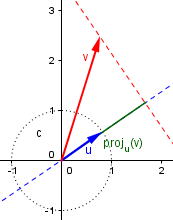Therefore, the dot product $$\mathbf{v}\cdot\mathbf{w}$$ can be thought of as the length of one, times the length of the projection of the other on it: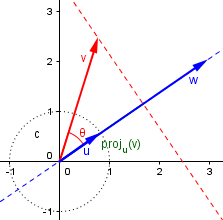Or we could say that the dot product is the projection of either unit vector on the other, multiplied by both lengths. This, in my mind, is the answer to Paul’s central question. But Doctor Anthony is right that this is not something whose importance would be initially obvious, leading one to make this definition.

Since it is just as easy to work with vectors in 3 dimensions as in 2 dimensions, you will find that most 3D geometry is done using vectors, and the dot product turns up in just about every problem you can think of; for example, finding the distance of a point from a plane or from a line, or the shortest distance between two lines in space, or the equation of a plane defined by three points. Some of these can also be solved using VECTOR products, but that is a more advanced concept.

It is the usefulness of the dot product, not what it represents in itself, that makes it worth defining. Because of the close connection of the dot product to the angle between vectors, many calculations that would involve complicated trigonometry can be done quickly by vectors.

In short, we don't set out to find the dot product. We set out to find angles between vectors, the component of a vector in some direction, the distance of a point from a line or plane, the equation of a plane, and so on and so on, and we use dot products in getting the answers to these questions. In a similar way, you don't multiply two numbers for the fun of it. You multiply numbers to answer some question which requires the technique of multiplication as an essential aid.

So what is the dot product? A simple tool that has powerful applications. It can be hard to picture, but its power comes from something deeper. (By the way, have you ever tried to explain what multiplication of numbers really is, when you are not just working with whole numbers? It can get complicated!)

## But where does that formula come from?

That answer begged the question of how the two “definitions” of the dot product, using components and using the angle, manage to come together. Later in 1998, we got this question:

Vector Angles: Prove A.B = |A||B|cosA

Hi Doctors,

I just finished my pre-calc class, and at the end we covered vectors, including this theorem:

A.B = |A||B|cosA
dot product = length(length)cos(angle between them).

If the proof isn't too complicated, what is the basis for that theorem? Any help would be appreciated.

Bryan

This assumes that the dot product has first been defined, presumably as the sum-of-products-of-components that Doctor Anthony started with, so that then a theorem can express it in a different form. Evidently it was presented in class just as an assertion, without a proof. Doctor Rick answered:

Hi, Bryan.

No, I don't think this proof is very complicated. Let's see if you agree.

To start with, let's draw a vector A with length a, making an angle alpha with the x-axis:

y
|
+....... + A
|       /:
|      / :
|     /  :
|   a/   :
|   /    : x = a*sin(alpha)
|  /     :
| /      :
|/)alpha :
+--------+------x
y = a*cos(alpha)

The x component of the vector is a*cos(alpha), and the y component is a*sin(alpha), from the right triangle I have drawn.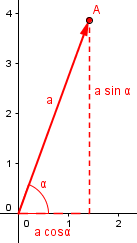Now make another vector B, of length b and making angle beta with the x-axis:

A = (a*cos(alpha), a*sin(alpha))
B = (b*cos(beta), b*sin(beta))

The dot product is now (just multiplying the x components and the y components, and adding them together)

A . B = a*b*cos(alpha)*cos(beta) + a*b*sin(alpha)*sin(beta)
= a * b * (cos(alpha)*cos(beta)+sin(alpha)*sin(beta))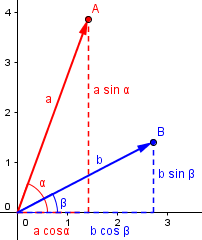All that's left is to remember the trigonometric identity,

cos(alpha - beta) = cos(alpha)*cos(beta) + sin(alpha)*sin(beta)

and we have

A . B = |A| * |B| * cos(alpha - beta)

But (alpha - beta) is the angle between the two vectors.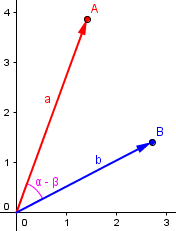So we started from the definition of the dot product as a sum of products of components (in two dimensions), and proved the cosine formula. This would have been harder to do in three dimensions, where a different approach would be needed.

### … or maybe it’s just a definition

There was a delay in that answer, and in between, Bryan had asked the same question just a little differently, indicating that the context was in fact three dimensions:

Hi Doctors,

In determining some angles in 3-D figures, I used the theorem:

A.B = |A||B|cosA    - pertaining to vectors

I was thinking this looked like the law of cosines, but I couldn't make the mental connection. Could you please help me to understand how the above theorem was derived?

The Law of Cosines is indeed a good idea; hold that thought!

Doctor Anthony answered, sort of reversing what he’d said in his previous answer:

This is not so much a 'theorem' as a 'definition'. You DEFINE the scalar products of two vectors to be the product:

a.b = |a| x |b| x cos of angle between a and b.

Following this definition you get the very convenient result that two vectors given in component form such as:

a = (x1,y1,z1)   b = (x2,y2,z2)  then the scalar product is:

a.b = x1.x2 + y1.y2 + z1.z2

This, of course, is the reverse approach from what we’ve said so far: If you define the dot product in terms of the angle, then you have to prove the component-wise form from that. The important thing is that both characterizations of the dot product can be shown to be equivalent. So let’s do that next.

## Finding the cosine

A couple months later, we had another similar question, to which we gave another two answers:

Deriving the Dot Product

I would like a very thorough explanation of how we derive the formula |u| |v| cos(x) = u.v, the dot product.

I have looked in several books and they merely write this as a given instead of explaining how and why we get this equation.

I need the formula so as to calculate the angle between two points on the surface of a sphere, but I want to understand what I am doing

The context suggests that this is another question about three dimensional vectors, but we’ll still be holding off on that. Note that Nick clearly has defined the dot product based on components, and treats this as a theorem; but he’s written it with the trigonometric form on the left.

Doctor Schwa replied first, with an approach that starts with the trig:

Good question!

There are books that do a better job of explaining this, but not a lot of them, so I'm not surprised you had trouble finding an answer.

The idea is to find the angle between two vectors, and show that it is u.v divided by |u| |v|, where I'll use "." to stand for the dot product and |u| to stand for the length of u.

Probably the best way to do this is to look at the angles made with the x-axis. We want to know the difference between the two angles, which I'll call theta_u and theta_v. Similarly I'll let the vector u have two components (x_u, y_u) and v be (x_v, y_v) (the standard notation is to use _ to denote subscripts).

x = theta_u - theta_v

Like Doctor Rick, he is going to work only in two dimensions; in effect, this will be the same proof approached in reverse. He starts with the angle-difference identity, as Doctor Rick ended with it:

I want to find cos(x), which is:

cos(theta_u - theta_v)
= cos(theta_u) cos(theta_v) + sin(theta_u) sin(theta_v)
= (x_u / |u|) (x_v / |v|) + (y_u / |u|) (y_v / |v|)

and simplifying, you get u.v / |u||v|

Very simple, it turns out, when you look at it the right way.

I'm just about to be teaching these ideas to my 11th grade class here at Gunn HS in Palo Alto so answering your question was a good reminder for me of what to emphasize. Thanks for asking it!

The fact that $$\displaystyle\cos(\theta_u) = \frac{x_u}{|\mathbf{u}|}$$ is equivalent to what we used before, that $$x_u = |\mathbf{u}|\cos(\theta_u)$$, or, in Doctor Rick’s notation, $$a_x = a\cos(\alpha)$$. This makes a nice, concise proof.

### Starting with |u||v|cosθ

Then Doctor Ken answered, again sticking to two dimensions:

Hi Nick,

Some books actually use |U|*|V|*Cos(X) as the definition of the dot product. There's another definition that's pretty common though; for two dimensional vectors, where U = (u1, u2) and V = (v1, v2), it is:

U.V = u1*v1 + u2*v2

For three-dimensional vectors, it's:

U.V = u1*v1 + u2*v2 + u3*v3

I bet you see the generalization.

So he has explicitly stated the important point: either can be taken as the definition; the goal is to prove that they are equivalent. He explicitly starts with the trig definition and derives the component form from it:

So let's see whether we can show that |U||V|Cos(X) = u1*v1 + u2*v2.  We'll write out the left side in terms of the coordinates of U and V, and then simplify. We'll do it in 2 dimensions, but the proof isn't very different in 3 dimensions.

Imagine a plane with two vectors:

U
V     |   /
\    |  /
\  | /
\|/
--------------------------------+----------------

Add the coordinates to the diagram:

U
V     |   /|
|\    |  / |
v2|  \  t /  |u2
|    \|/   |
--------------------------+-----O----+-----------X
v1     u1

Angle t is the angle from U to V. It equals the angle from the x-axis to V, minus the angle from the x-axis to U.  Let's call these angles XOV and XOU. So we have:

|U||V|Cos(x) = |U||V|Cos(t)
= |U||V|Cos(XOV - XOU)
= |U||V|[Cos(XOV)Cos(XOU) + Sin(XOU)Sin(XOV)]
= |U||V|[(v1/|V|)(u1/|U|) + (v2/|V|)(u2/|U|)]
= |U||V|[(v1u1 + v2u2)/(|V||U|)]
= v1u1 + v2u2

I’ve added some labels to his diagram, and called the angle t, to make it easier to follow.

You should be able to see how this is the same as our previous proofs, just given a different twist.

Make sense? Now, to make sure you understand it, since your problem is in 3 dimensions, why don't you try to derive the same result for 3-dimensional vectors? If you're slick, you can actually use the 2-dimensional result (hint: there's a plane that contains the two vectors and the origin).

I haven’t found a nice way to extend this method of proof to three dimensions; when we have been asked about that comment, we have generally offered the method given in the next answer, which extends easily to that case. It is possible that the extension of the angle-difference method requires more advanced knowledge of either vectors or geometry than I want to assume. (If anyone finds a clear way to do it, let me know!) So let’s finish with the most powerful proof:

## A proof using the Law of Cosines

In 2006, Sheri asked it this way:

How Does the Dot Product of Two Vectors Work?

I'm a senior in high school studying advanced math and we are now studying about the dot product.  My question is, how do we describe the identity function in terms of the dot product formula?  Our teacher was talking about how they're related but I'm absolutely confused!  I don't even know what the dot product is about and how does multiplying two vectors give you the angle they form together?

I’m not sure what Sheri means by “the identity function”; she may mean the angle-sum identity used in all our answers so far! If so, she got something different. Doctor Jerry replied:

Hello Sheri,

Thanks for writing to Dr. Math.

I'll be referring to this image: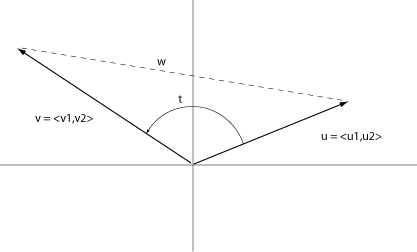The vectors u and v are given, t is the angle between them (typing "t" is easier than typing "theta"), and w is the length of the line joining the tips of u and v.  The components of u and v are u1, u2, v1, and v2.  You may usually write u = u1*i + u2*j.

In the diagram, Doctor Jerry used the standard notation for vectors, $$\langle a, b\rangle$$. In the form we have been using here, the vectors are $$\mathbf{u} = (u_1, u_2)$$ and $$\mathbf{v} = (v_1, v_2)$$. We’ve seen previously that they can be written using unit basis vectors as $$\mathbf{u} = u_1\mathbf{i} + u_2\mathbf{j}$$ and $$\mathbf{v} = v_1\mathbf{i} + v_2\mathbf{j}$$.

The length of w can be calculated using the law of cosines.  For this, notice that the coordinates of the tips of u and v are (u1,u2) and (v1,v2).  From the law of cosines and letting sqrt(u1^2 + u2^2) = |u|, the length of u, and sqrt(v1^2 + v2^2) = |v|, the length of v, we see that:

w^2 = |u|^2 + |v|^2 - 2*|u|*|v|*cos(t)

Because w^2 = (u1-v1)^2 + (u2-v2)^2, we see that:

(u1-v1)^2 + (u2-v2)^2 =  |u|^2 + |v|^2 - 2*|u|*|v|*cos(t)

Many people, when they first see the formula for the dot product, recognize its similarity to the Law of Cosines, so this is a natural thing to try.

If we now replace |u|^2 and |v|^2 by u1^2 + u2^2 and v1^2 + v2^2 we find:

(u1-v1)^2 + (u2-v2)^2 = u1^2 + u2^2 + v1^2 + v2^2 - 2*|u|*|v|*cos(t)

Expanding the left side and simplifying:

u1*v1 + u2*v2 = |u|*|v|*cos(t)

The left side is usually given the name "dot product" and written as "u.v", so:

u.v = |u|*|v|*cos(t)

As I hope you find out, this is a very useful result.

This is easy to extend to three dimensions:

$$|(u_1,u_2,u_3)-(v_1,v_2,v_3)|^2=|(u_1,u_2,u_3)|^2+|(v_1,v_2,v_3)|^2-2|\mathbf{u}| |\mathbf{v}|\cos\theta$$

$$(u_1-v_1)^2+(u_2-v_2)^2+(u_3-v_3)^2=u_1^2+u_2^2+u_3^2+v_1^2+v_2^2+v_3^2-2|\mathbf{u}| |\mathbf{v}|\cos\theta$$

$$u_1^2-2u_1v_1+v_1^2+u_2^2-2u_2v_2+v_2^2+u_3^2-2u_3v_3+v_3^2=u_1^2+u_2^2+u_3^2+v_1^2+v_2^2+v_3^2-2|\mathbf{u}| |\mathbf{v}|\cos\theta$$

$$- 2u_1v_1-2u_2v_2-2u_3v_3=- 2|\mathbf{u}| |\mathbf{v}|\cos\theta$$

$$u_1v_1+u_2v_2+u_3v_3=|\mathbf{u}| |\mathbf{v}|\cos\theta$$

$$\mathbf{u}\cdot\mathbf{v}=|\mathbf{u}| |\mathbf{v}|\cos\theta$$

### 4 thoughts on “Multiplying Vectors I: The Scalar Product”

This site uses Akismet to reduce spam. Learn how your comment data is processed.